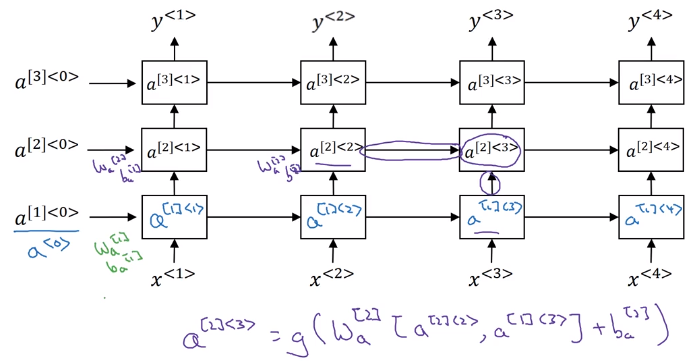# week1

Created Friday 02 February 2018

### Why sequence models

examples of seq data (either input or output):

• speech recognition
• music generation
• sentiment classification
• DNA seq analysis
• Machine translation
• video activity recognition
• name entity recognition (NER)

→ in this course: learn models applicable to these different settings.

### Notation

motivating example: NER (Each word: whether the word is part of a person's name.)

• `x^<t>` / `y^<t>`: t-th element in input/output sequence.
• `X^(i)`: i-th training example
• `T_x^(i)`: length of i-th training example sequence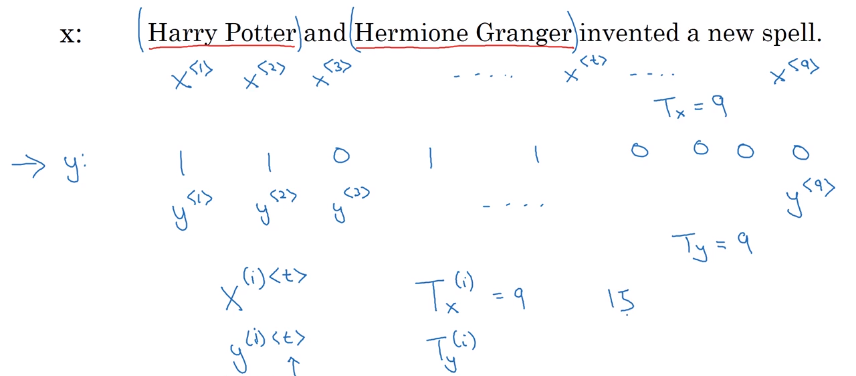how to repr each words in a sentences (x)

• vocabulary: list of ~10k possible tokens (+"" for unknown words)
• one-hot repr for each word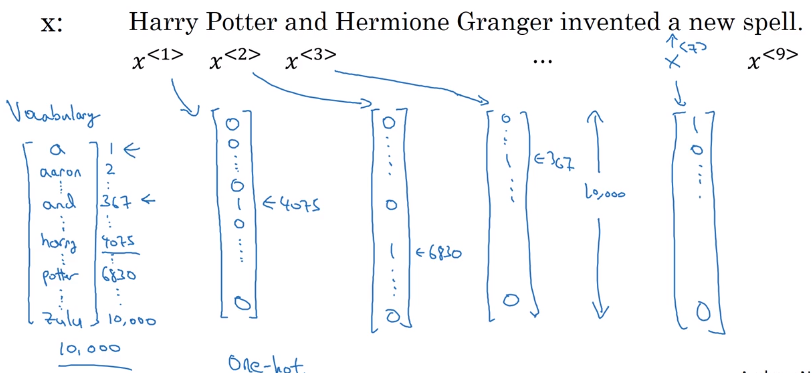### Recurrent Neural Network Model

why not a standard NN

• input/output are of different length (padding might not be a good representation)
• doesn't share features learned across different positions in text

→ using a better representation helps to reduce number of parameters.

#### RNN

motivation example: output length = input length

• for each input `x<t>`, → feed to RNN → compute activation(hidden state) `a<t>`, and output `y<t>`,
• `y<t>=f(a<t>)`, `a<t>=f(x<t>, a<t-1>)`, i.e. a depends on previous state and current input.
• parameters `W_ax` / `W_aa` / `W_ya` are shared across all time steps.

`y<t>` depends on `x<1>...x<t>`, limit: only depend on previous words ("unidirectional").

Unrolled diagram: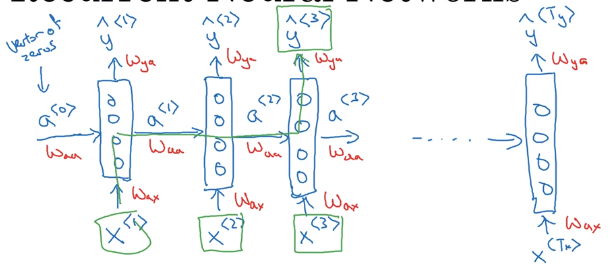Or drawing a recurrent loop (harder to understand than unrolled version):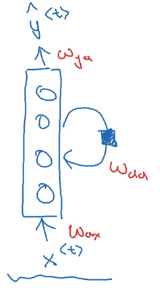#### RNN Forward prop

formula to calculate `a<t>` and `y<t>`:
a = g(W_aa * a + W_ax * x + b_a)
y = g(W_ya * a + b_y)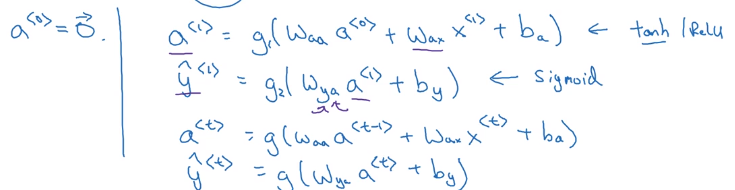Simplified annotation: stack `a<t-1>` and `x<t>`, `W_a = [W_aa, W_ax]`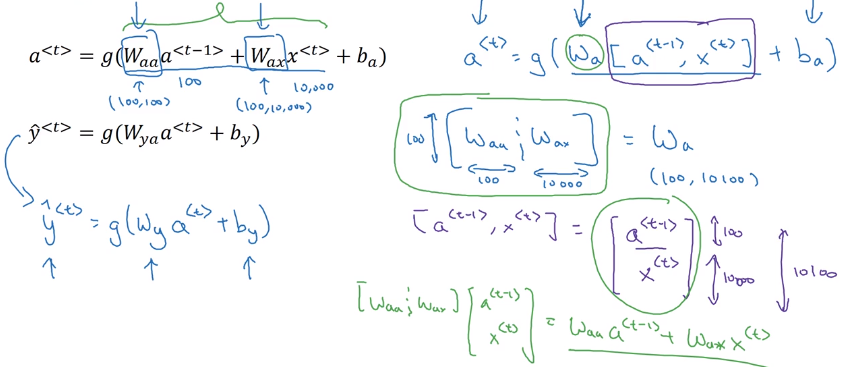### Backpropagation through time

Parameters: `W_y` and `W_a`
loss function: log loss at each timestep (assume predictions y are binary)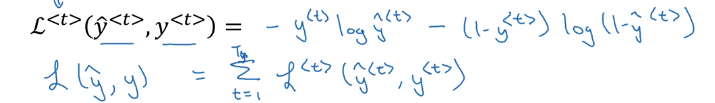→ backporp through the computation graph: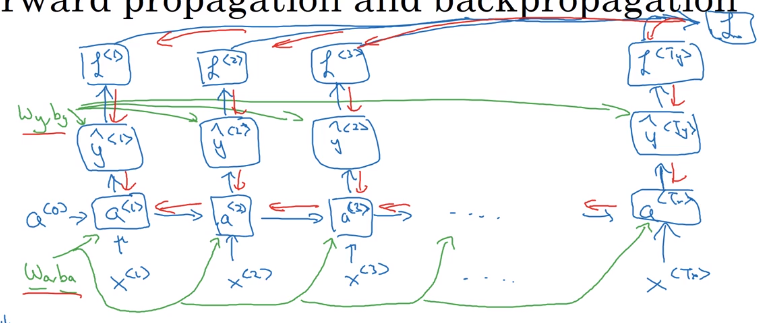### Different types of RNNs

many-to-many:

• T_x = T_y, one prediction per timestep.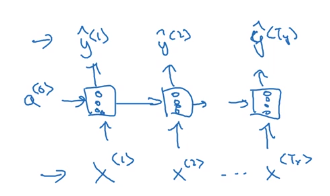• T_x != T_y, e.g. machine translation

having a encoder and a decoder: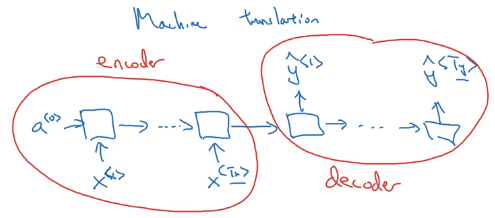many-to-one:
e.g. sentence classification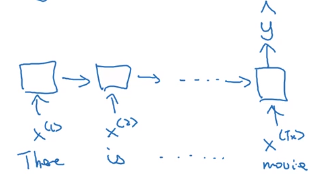one-to-many:
e.g. music generation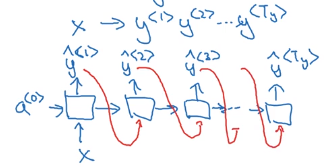### Language model and sequence generation

Language model
motivation example: speech recognition,
"The apple and the pear salad" VS "The apple and the pair salad"
language model: give probability of a sentence P(sentence).

Building language model with RNN
Training set: large corpus of text.

• tokenize
• vocabulary size
• unknow word "".

RNN for seq generation

• output `y<t>`: softmax of probability for each word.
• `y<t+1>`: make prediction given the correct previous word
• like this predict one word at a time.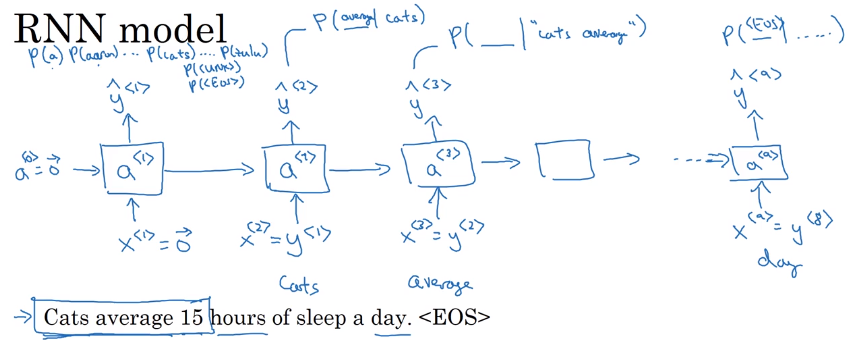Loss function: cross entropy (actual word VS probability of this word) at each timestep.### Sampling novel sequences

Get a sense of what's learned: sample nouvel seqs.
From training, the RNN has a distribution of sequences `P(y<t> | y<1...t-1>)`.
In sample: let the model generate sequences (`np.random.choice`):

• feed previously gen word as input to next step
• include token in vocab to finish
• reject tokens

char-level language model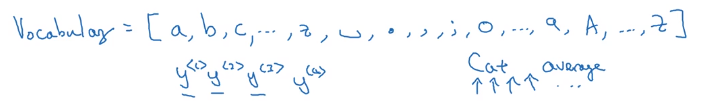• pro: no token
• con: much longer sequences, more expensive to train.

long-range dependencies are hard to capture:
e.g. "the cat ........ was full" VS "the cats ...... were full"

this is due to vanishing gradients: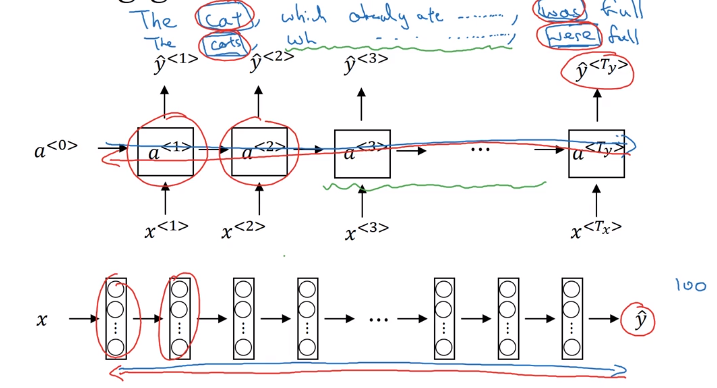### Gated Recurrent Unit (GRU)

Modification of RNN to capture long range dependencies.

Visualization of a RNN unit: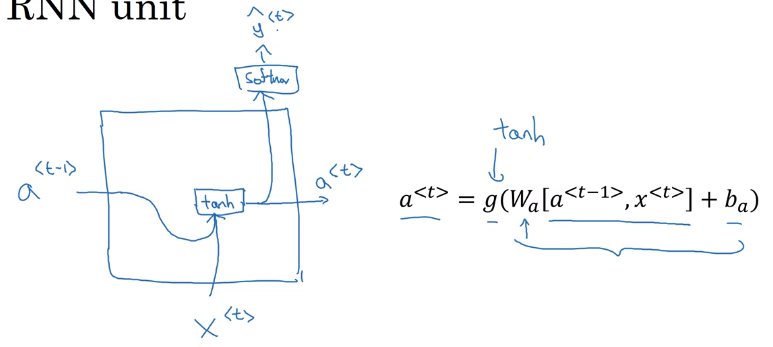(simplified) GRU

• Extra memory cell: `c<t>=a<t>`, (replaces output activation).
• Candidate value of c: `c_tilde<t>=tanh(Wc * [c<t-1>, x<t>] + b_c)`
• Gate (between 0 and 1, conceptually consider it as binary):

`Gamme_u = sigmoid(W_u * [c<t-1>, x<t>] + b_u)`
subscript "u" stands for "update", i.e. whether we want to update current memory cell

• Actual value of `c<t> = Gamme_u * c_tilde<t> + (1-Gamma_u) * c<t-1>` (`*` is element-wise multiplication)

i.e. `c<t>` can be conserved for long range before being updated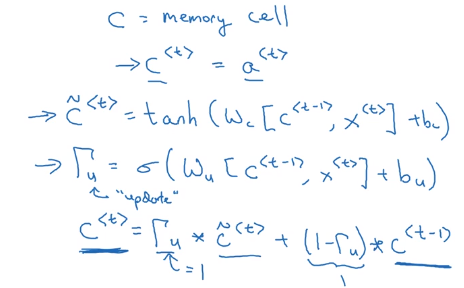Visualization of GRU unit (maybe equations are more understandable...):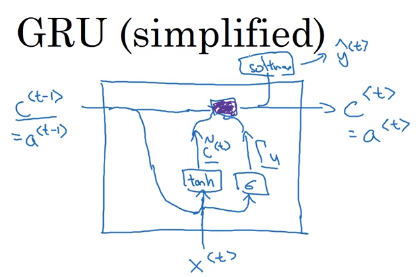full GRU
for candidate `c_tilde<t>`, add one more gate `Gamme_r`: controlling how much `c<t-1>` contributes to `c_tilde<t>`("r" for "relevance", i.e. how relevant `c<t-1>` is for `c_tilde<t>`)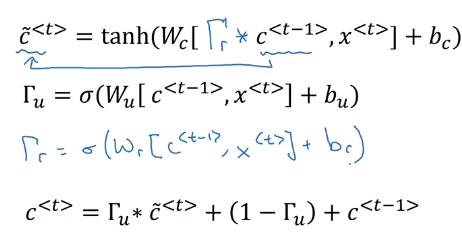### Long Short Term Memory (LSTM)

More powerful and general version of GRU.

• output `a<t>` no longer equals to memory cell `c<t>` (but a gated version of it, see below)
• candidate `c_tilde<t>` depends on `a<t-1>` instead of `c<t-1>`
• two update gates: `Gamma_u` (update gate) and `Gamma_f` (forget gate)
• output gate: `Gamma_o`
• value of `c<t> = Gamma_u * c_tilde<t> + Gamma_f * c<t-1>`
• value of `a<t> = Gamma_o * c<t>`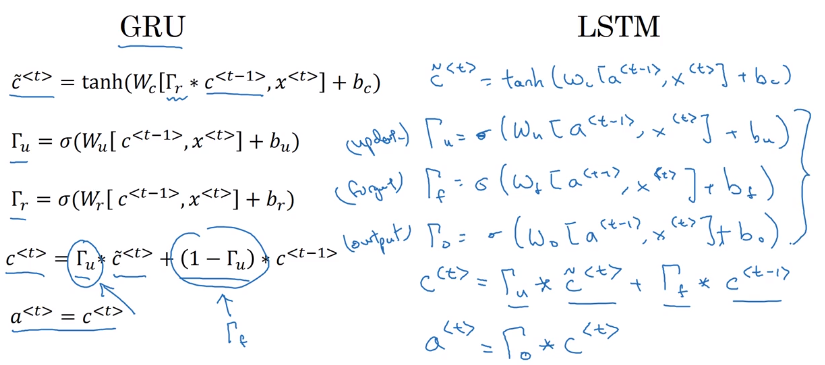Visualization:Intuition: c can be kept for long time if gates are set properly.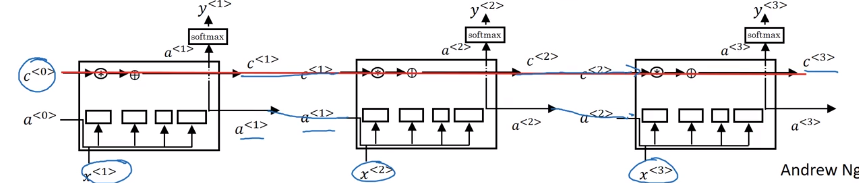Variant: let the gates depend on c as well ("peephole connection")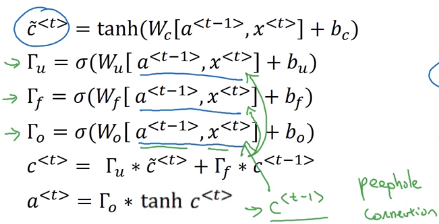GRU vs LSTM

• LSTM is proposed earlier
• GRU as a simplified version of LSTM
• GRU easier to train larger NN (2 gates instead of 3)
• LSTM more powerful, recommended default choice to try

### Bidirectional RNN

Getting information from the future.
motivation example: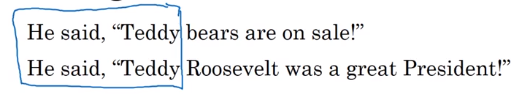Bidirectional RNN (BRNN)

• forward and backword recurrent components
• computation graph is still acyclic
• at t, both information from the past and the future are passed in
• BRNN with LSTM blocks are typically the first thing to try in NLP problems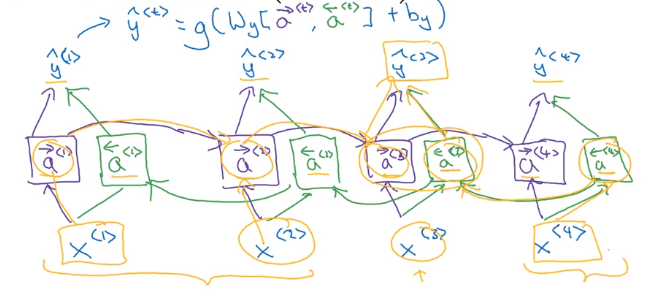### Deep RNNs

Complex NN: stack multiple RNNs (having 3 RNN layers is already a lot).0
notation: `a[l]<t>` for activation in layer `l` and time `t`.